# VSWR

The voltage standing wave ratio (VSWR) is the ratio of the peak amplitude to the minimum amplitude of a standing wave. An ideal antenna has a VSWR of 1 (no reflected power), but the VSWR is often higher. The VSWR can be calculated with the reflection coefficient or with the forward power and the reflected power. The reflection coefficient describes the power that is reflected from the antenna. The VSWR can be used to calculate the return loss and mismatch loss.

### Formulas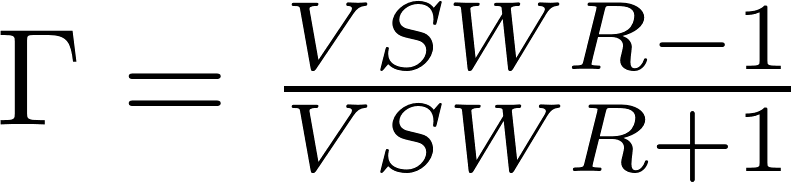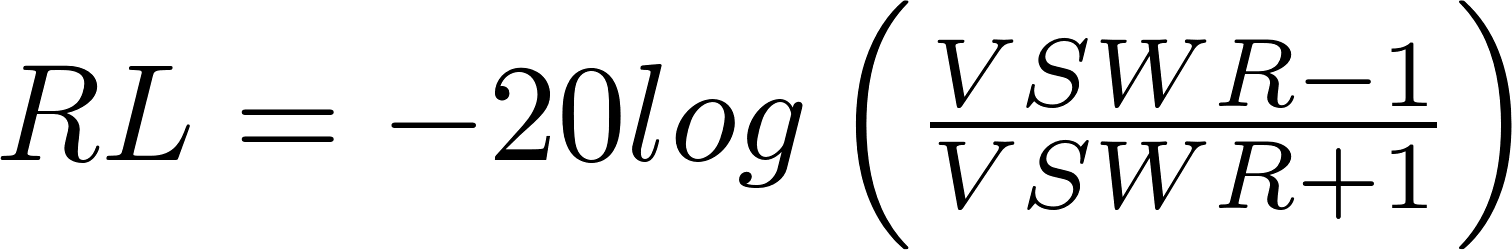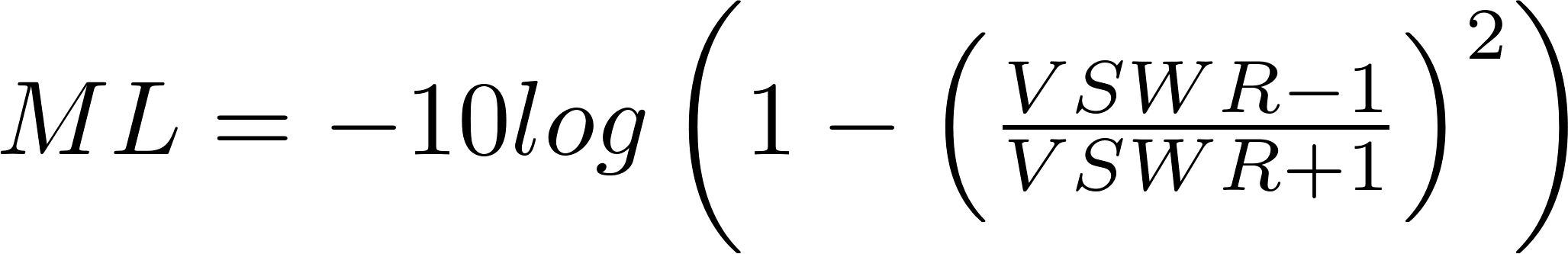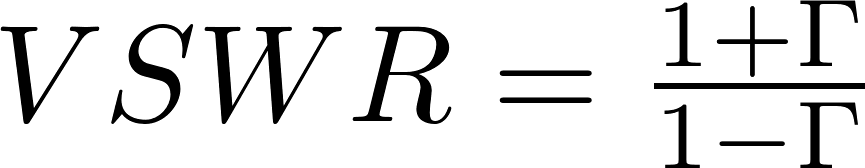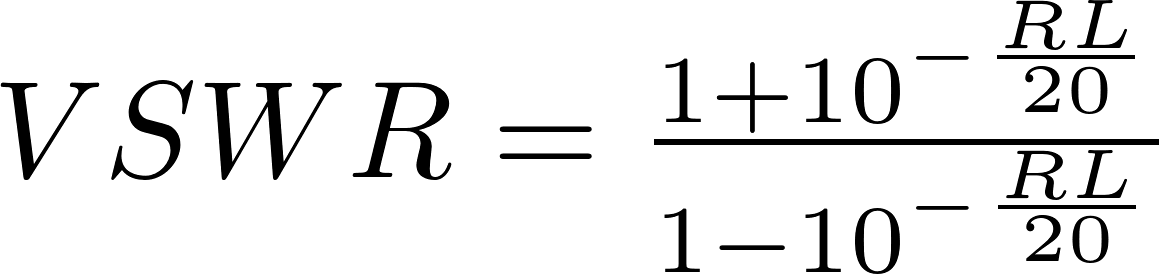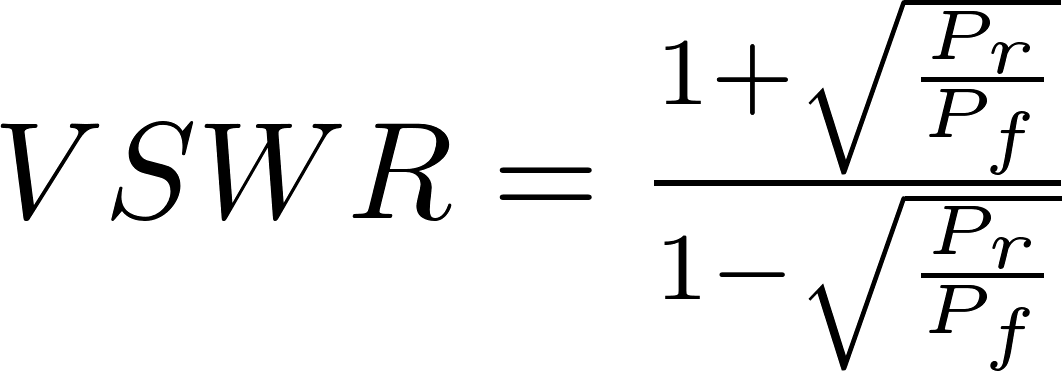RL is the symbol for return loss and is measured in decibel (dB).
ML is the symbol for mismatch loss and is measured in decibel (dB).
RC is the reflection coefficient.
P f is the symbol for forward power and is measured in watt (W).
P r is the symbol for reflected power and is measured in watt (W).